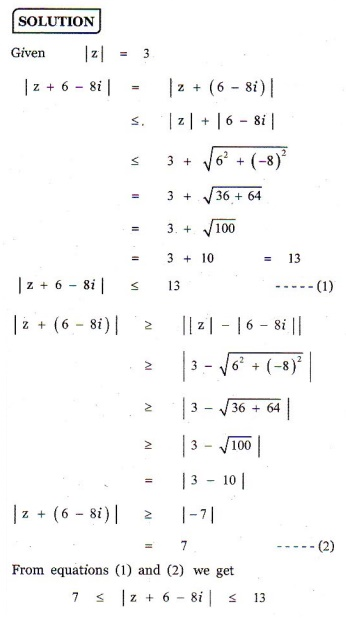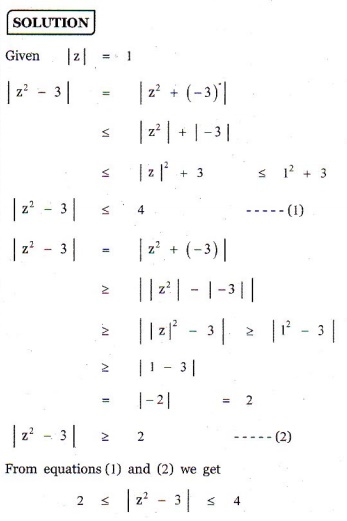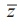Home | | Maths 12th Std | Exercise 2.5: Modulus of a Complex Number

# Exercise 2.5: Modulus of a Complex Number

Maths Book back answers and solution for Exercise questions - Mathematics : Complex Numbers: Modulus of a Complex Number: Problem Questions with Answer, Solution

EXERCISE 2.5

1. Find the modulus of the following complex numbers2. For any two complex numbers z1 and z2 , such that |z1| = |z2| = 1 and z1z2 ã  -1, then show that [z1  + z2] / [1+ z1z2] is a real number.3. Which one of the points10 - 8i, 11 + 6is closest to1+ .4. If | |= 3 , show that 7 ãÊ | + 6 ã8| ãÊ13 .5. If  |z| = 1, show that 2 ãÊ |z2 ã 3| ãÊ 4.6. If= 2 , show that the greatest and least value of | z | are ã3 +1 and ã3 - 1 respectively.7.  If z1, z2, and z3 are three complex numbers such that |z1| = 1, |z2| = 2, |z3| = 3 and |z1 + z2 + z3| = 1 , show that |9z1z2 + 4z1z3 + z2z3| = 6.8. If the area of the triangle formed by the vertices ziz , and iz is 50 square units, find the value of |z|.9. Show that the equation z3 + 2= 0 has five solutions.10. Find the square roots of (i) 4 + 3i  (ii) -6 + 8(iii) -5 -12.